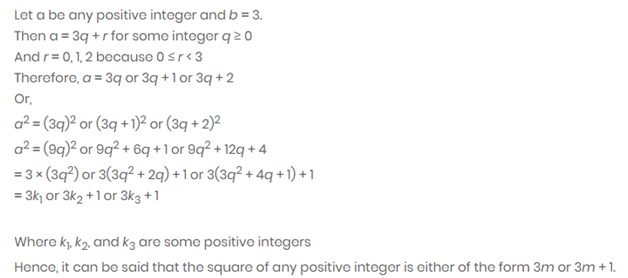• +91 9971497814
• info@interviewmaterial.com

# Real Numbers Ex-1.1 Interview Questions Answers

### Related Subjects

Question 1 : Use Euclid’s division algorithm to find the HCF of:

i. 135 and 225

ii. 196 and 38220

iii. 867 and 225

Solutions:

Q i.    135 and 225

i. 135 and 225

As you can see, from the question 225 isgreater than 135. Therefore, by Euclid’s division algorithm, we have,

225 = 135 × 1 + 90

Now, remainder 90 ≠ 0, thus again usingdivision lemma for 90, we get,

135 = 90 × 1 + 45

Again, 45 ≠ 0, repeating the above step for45, we get,

90 = 45 × 2 + 0

The remainder is now zero, so our method stopshere. Since, in the last step, the divisor is 45, therefore, HCF (225,135) =HCF (135, 90) = HCF (90, 45) = 45.

Hence, the HCF of 225 and 135 is 45.

Q ii.     196 and 38220

ii. 196 and 38220

In this given question, 38220>196,therefore the by applying Euclid’s division algorithm and taking 38220 asdivisor, we get,

38220 = 196 × 195 + 0

We have already got the remainder as 0 here.Therefore, HCF(196, 38220) = 196.

Hence, the HCF of 196 and 38220 is 196.

Q iii.    867 and 225

iii. 867 and 225

As we know, 867 is greater than 225. Let usapply now Euclid’s division algorithm on 867, to get,

867 = 225 × 3 + 102

Remainder 102 ≠ 0, therefore taking 225 asdivisor and applying the division lemma method, we get,

225 = 102 × 2 + 51

Again, 51 ≠ 0. Now 102 is the new divisor, sorepeating the same step we get,

102 = 51 × 2 + 0

The remainder is now zero, so our procedurestops here. Since, in the last step, the divisor is 51, therefore, HCF(867,225) = HCF(225,102) = HCF(102,51) = 51.

Hence, the HCF of 867 and 225 is 51.

Question 2 :

Show that any positive odd integer is of theform 6q + 1, or 6q + 3, or 6q + 5, where q is some integer.

Answer 2 :Question 3 :

An army contingent of 616 members is to marchbehind an army band of 32 members in a parade. The two groups are to march inthe same number of columns. What is the maximum number of columns in which theycan march?

Solution:

Given,

Number of army contingent members=616

Number of army band members = 32

By Using Euclid’s algorithm to find their HCF,we get,

Since, 616>32, therefore,

616 = 32 × 19 + 8

Since, 8 ≠ 0, therefore, taking 32 as newdivisor, we have,

32 = 8 × 4 + 0

Now we have got remainder as 0, therefore, HCF(616, 32) = 8.

Hence, the maximum number of columns in whichthey can march is 8.

Question 4 :

Use Euclid’s division lemma to show that thesquare of any positive integer is either of the form 3m or 3m + 1 for someinteger m.Question 5 :

Use Euclid’s division lemma to show that thecube of any positive integer is of the form 9m, 9m + 1 or 9m + 8.

Solution:

Let x be any positive integer and y = 3.

By Euclid’s division algorithm

Now

x = 3q+r, where q≥0 and r = 0, 1, 2, as r ≥ 0and r < 3.

Then, putting the value of r

We get,

x = 3q  or  x = 3q + 1    or      x = 3q + 2

Now, by taking the cube of all the three aboveexpressions.

Case (i): Whenr = 0

Then,

x2= (3q)3 = 27q3=9(3q3)= 9m; where m = 3q3

Case (ii): Whenr = 1

Then,

x3 = (3q+1)3 =(3q)+13+3×3q×1(3q+1) = 27q3+1+27q2+9q

Taking 9 as common above factor

We get,

x= 9(3q3+3q2+q)+1

Putting = m

We get,

Putting (3q3+3q2+q) = m,we get ,

x3 = 9m+1

Case (iii): When r = 2

Then,

x3 = (3q+2)3

=(3q)3+23+3×3q×2(3q+2)

=27q3+54q2+36q+8

Taking 9 as common above factor

We get,

x3=9(3q3+6q2+4q)+8

Putting (3q3+6q2+4q) = m

We get ,

x3 = 9m+8

Therefore, from all the three cases explainedabove, it is proved that the cube of any positive integer is of the form 9m, 9m+ 1 or 9m + 8.

Todays Deals### Real Numbers Ex-1.1 Contributorskrishan

Name:
Email:

# Latest News# 9000 interview questions in different categories